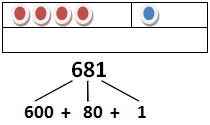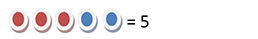Decomposition of Numbers

My Definition

Key Characteristics

The following are true of decomposition of numbers:

• To decompose a number or set is to break it apart or separate it into two or more parts.

• To decompose numbers can mean to determine a sum positive rational numbers that give a specific value.

• To decompose numbers can also mean to factor a composite number.

Note: In Algebra II and subsequent coursework, the verb "decompose" refers to recognition of functions that were combined to make a new one.

Example

Decomposing sets or numbers.

15 can be decomposed into numerous sums:

10 + 5

10 + 2 + 3

8 + 7

How might you decompose 23? 427? 58?

Five has a part that is four and a part that is one.Non-example

Joining sets or numbers together, such as three red counters and two blue counters, combine to make a set of five counters.Combining numbers through addition reflects composing a number.

300 + 50 + 8 = 358

Click on the link below to further study examples of composition of numbers.

Composition of Numbers ActivityTEKS: K(2)(I), 1(2)(B), 1(3)(D), 2(2)(A), 3(2)(A), 3(3)(D), 4(3)(B)